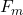## The picture tube in an old black-and-white television uses magnetic deflection coils rather than electric deflection plates. Suppose an elec

Question

The picture tube in an old black-and-white television uses magnetic deflection coils rather than electric deflection plates. Suppose an electron beam is accelerated through a 498-V potential difference and then through a region of uniform magnetic field 1.00 cm wide. The screen is located 10.4 cm from the center of the coils and is 49.0 cm wide. When the field is turned off, the electron beam hits the center of the screen. Ignoring relativistic corrections, what field magnitude is necessary to deflect the beam to the side of the screen?

in progress 0
5 months 2021-08-18T01:27:50+00:00 1 Answers 11 views 0

B = 0.368 T

Explanation:

Let’s solve the problem by parts, let’s start by finding the speed of the electron beam

F = ma

a = e E / m

The field and the electric potential are related

V = – E x

E = -V / x

a = e/m  V/x

We use kinematics

v² = v₀² + 2 a x

v² = 0+ 2 a x

v = √2 (e V / xm) x

v = √ 2 e V / m

v = √ (2 1.6 10⁻¹⁹ 498 / 9.1 10⁻³¹)

v = √ (175.12 10¹²)

v = 13.23 10⁶ m / s

Now the electrons enter the magnetic field, the modulus of its speed does not change, let’s find the time to reach the screen

v = x / t.

t = x / v

t = 0.01 / 13.23 10⁶

t = 7.56 10⁻¹⁰ s

At this same time you have to get to the side of the screen y = 0.245 m

y = v₀ t + ½ a t²

The initial speed on this axis is zero, because when we turn off the magnetic field the electrons reach the center of the screen

a = 2y / t²

a = 2 0.245 / (7.56 10⁻¹⁰)²

a = 8.57 10¹⁷ m / s²

We use Newton’s second law= m a

q v B = m a

B = m a / q v

B = 9.1 10⁻³¹  8.57 10¹⁷ / (1.6 10⁻¹⁹ 13.23 10⁶)

B = 0.368 T